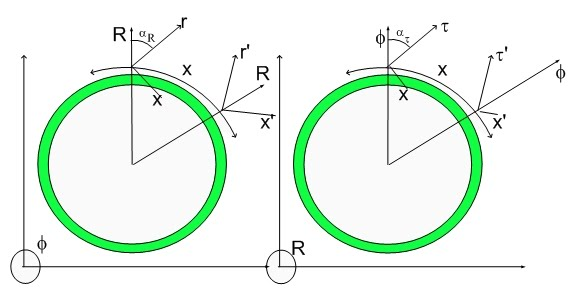The miniBooNE Experiment

If I had a Pion for every time I corrected Science I would be a very rich man...:)

The miniBooNE experiment was created to confirm the Neutrino Oscillatory Model, that is, it was designed to see neutrinos metamorphose themselves as a function of distance traversed from point they were created.

After reading this posting you will realize why I don't believe they will see an oscillation, despite the fact that I created the appropriate relationship between the three neutrino states...:)

First, I heard or read the there were missing electron neutrinos from fusion reactions in the Sun and that was the reason for the Neutrino Oscillation Model proposition. Someone got a Nobel Prize for that idea..:) - don't quote me on that...:) I haven't kept in touch with the
Nobelists lately...

At first, I thought that that was a stupid idea. The masses of the three neutrinos alledgedly involved in this menage a trois were extremely different.

Fermion Symbol Mass
Generation 1 (electron)
Electron neutrino 2.2 eV
Electron antineutrino 2.2 eV
Generation 2 (muon)
Muon neutrino 170 keV
Muon antineutrino 170 keV
Generation 3 (tau)
Tau neutrino 15.5 MeV
Tau antineutrino 15.5 MeV

There was no way that a punny electron neutrino would convert into a gigantic Tau neutrino for any measurable length of time (larger than the uncertainty principle associated with that mass or energy difference). I wouldn't expect that event to be measurable.

Initiallly, I recognized that the Tau Neutrino which was considered to be so fat could be just an intermediate Fat State... a Glutonic Phase of the Tau Neutrino that he would just as well want to forget...:)

Let's review the origin of that Glutonic State (Tau Neutrino Fat State).

The Tau Lepton decays accordingly to the branching ratio:

17.84% for decay into a tau neutrino, electron and electron neutrino
17.36% for decay into a tau neutrino, muon and muon neutrino

or into
3 Pions plus a Tau Neutrino. The other relevant reference is here

The mass confusion is due to the fact that the three decay paths mentioned would result in three different Glutonic States of the Tau Neutrino..:)

Let's consider first the reduced representation of the Tau Neutrino and its relationship with the Muon and Electron Neutrinos. Their diagrams is shown below: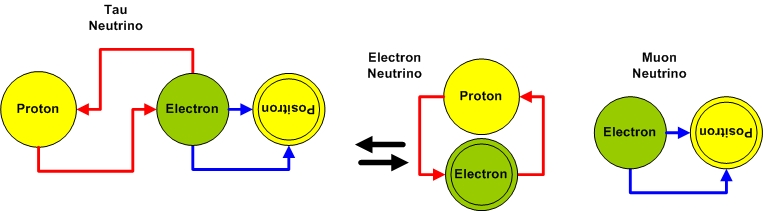This diagrams are easily associated with their state diagram shown below: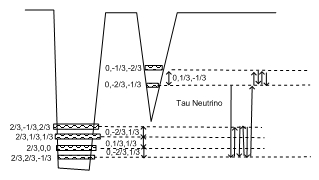where one can easily see the Proton State coherences (Electron Neutrino - which is a sum of two Electron-Proton Transmutation chords) and Electron State coherences (Muon Neutrino - which is a sum of two Electron-Positron Transmutation chords).

The Tau Lepton decaying into three Pions gives away its slimmest Tau Neutrino.
Below is the Tau Lepton: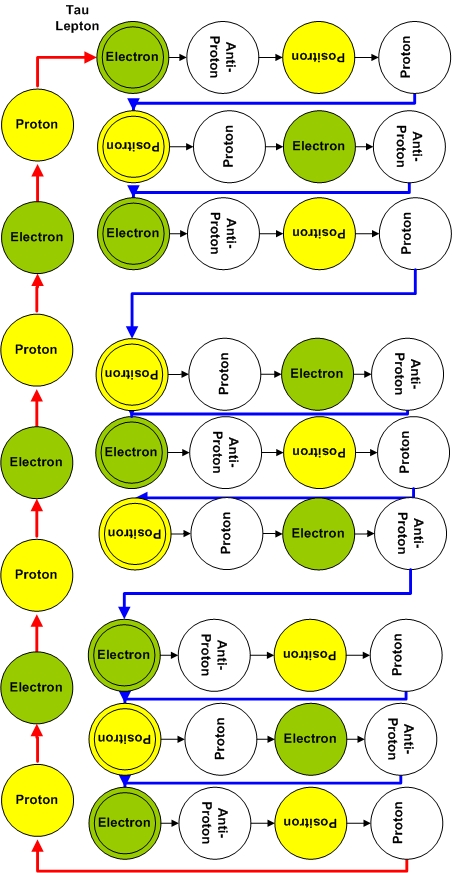which decays into: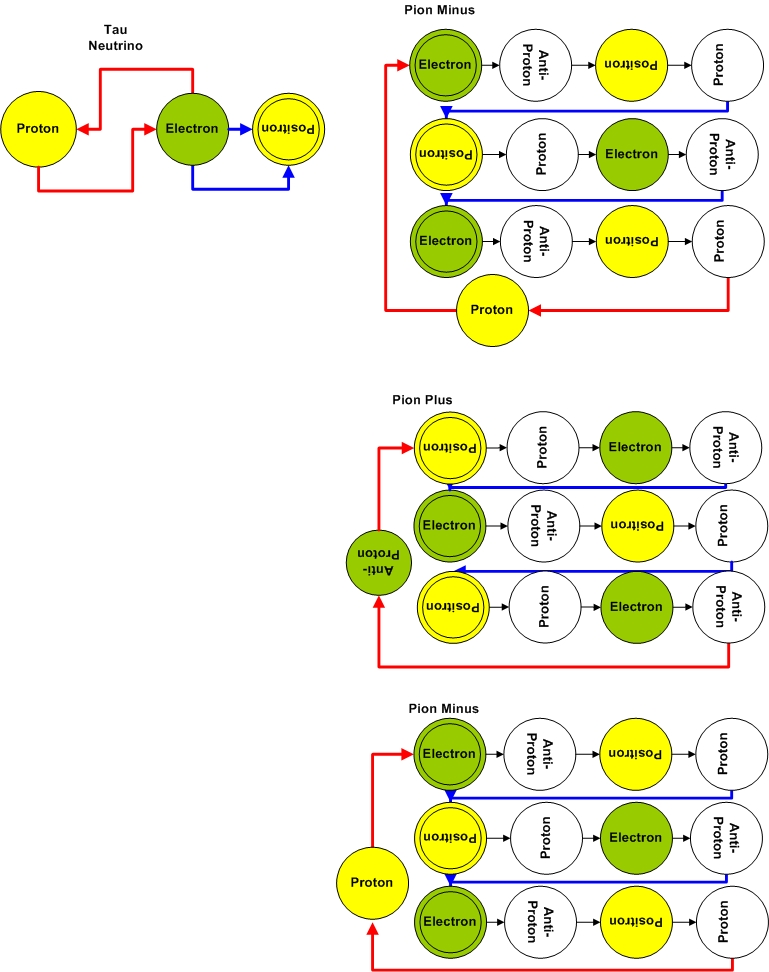Of course, the Electron-Proton transmutation chord which corresponds to a spatial rotation in the 3D space (hypersurface) would become a Positron-Antiproton transmutation chord if applied to an initial positronic state...:)

It is easy to see that if one breaks apart the Tau Lepton along the two other branches, the resulting Tau Neutrino will carry the leftover mass. Let's consider the first branch:
17.84% for decay into a tau neutrino, electron and electron neutrino

Here are the products from the Tau Minus Lepton decay (tau neutrino, electron and electron neutrino):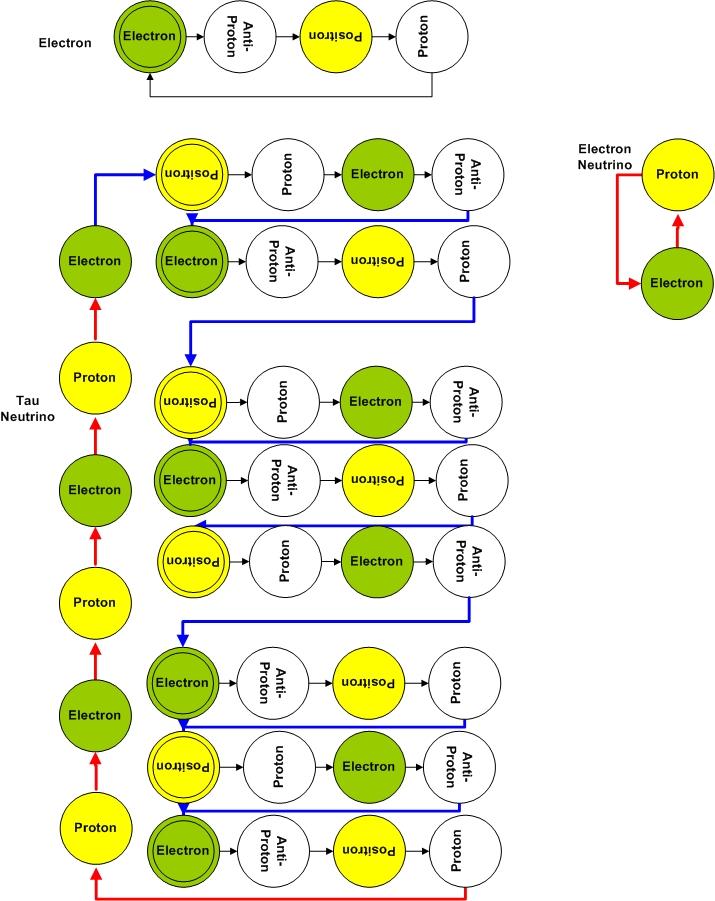as you can see this is a Fatter Tau Neutrino. The extra energy is later released when Electrons and Positrons intra-coherences annihilate each other. Of course, as the coherence simplifies itself, energy is stored in linear and angular momentum. A simplied coherence rotates in 3D faster (complex coherences have helicity due to pseudo rotation).

The other decay pathway will result in another Tau Neutrino.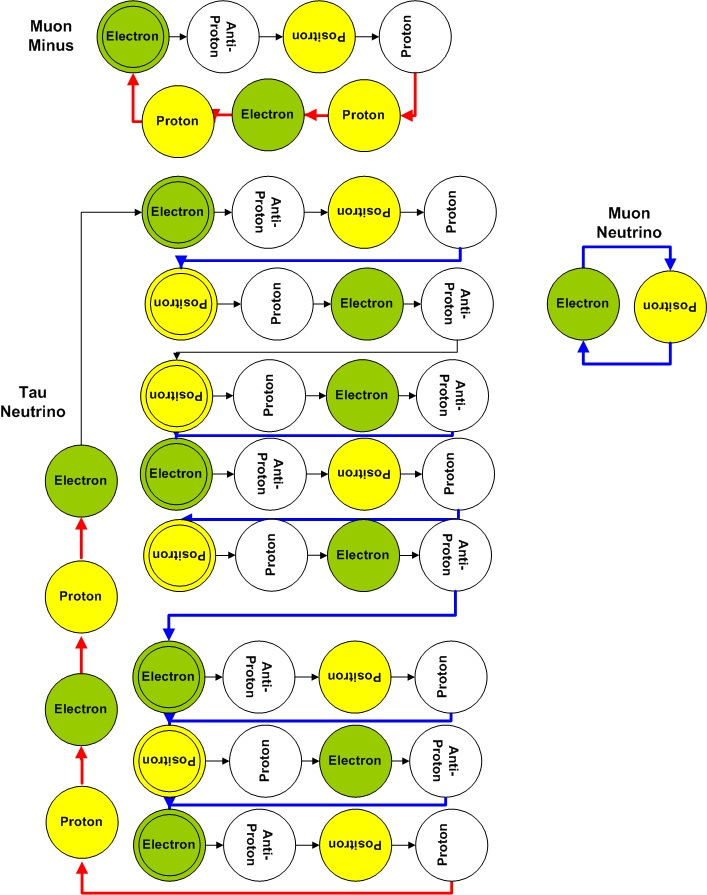As you can see the transmutation chords are preserved, although the number of transmutation chords is not conserved within the Tau Neutrino and thus these two Glutonic States have different masses...:)

The second part of the puzzle is an experiment that doesn't produce the number of neutrinos the current standard model expected. The experiment is simply the decay of a Pion>>:)

A Pion Minus decays into an Muon Minus and a muon neutrino.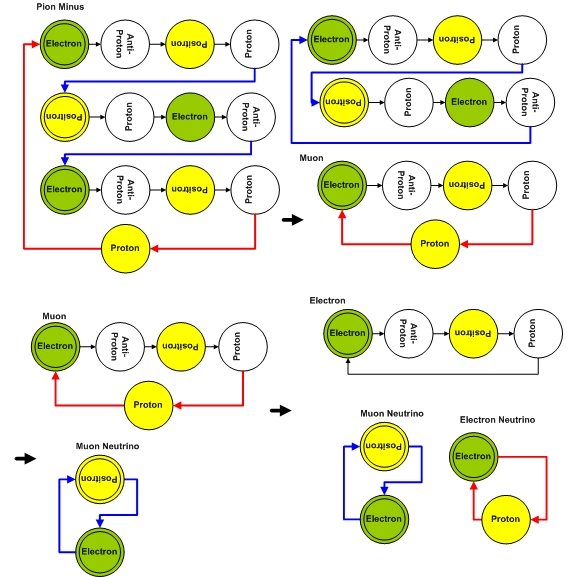On the other hand, a Muon Minus decays into an electron, a muon neutrino and an electron neutrino >>>> Hence the Pion Minus has to decay into an electron, two muon neutrinos and one electron neutrino.. That is obvious..:)

Experimental evidence indicates that the ratio between muon and electron neutrinos is closer to one..>:)

Of course, this is what one would have if one were to understand the Universe in a simpleton manner, that is, in terms of particles...

In terms of coherences the perspective is different. The simple answer is that a Pion Minus also decays into an electron, a muon neutrino and an electron neutrino directly...:)

How could that be...:) One would expect that the itsy bitsy parts of the pion to be preserved..:) quarks etc...

The image is different if one considers a Fourier view (coherence view) of particles. In that case, the muon neutrino is equivalent to a rotation of the electron state around in the 3D space. This part of the coherence repeats together with the coherence for the lifetime of the Pion. If the pion components (electron and positron) annhihilate themselves such that the two electron-positron transmutation chords become sequential that doesn't change the nature of that chord. It is still a muon neutrino. This means that two (many) muon neutrinos intra-chord is the same as one single neutrino ejected.

Below is the Pion Minus decaying into an electron and a single muon neutrino and an electron neutrino.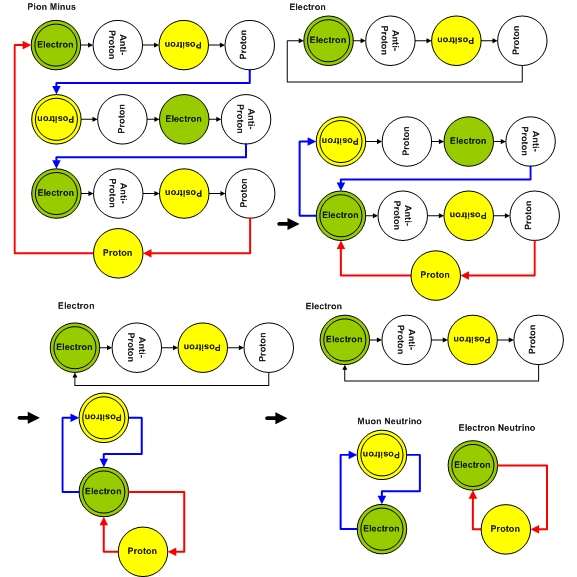This is the simple answer for the neutrino problem.

Cheers,

MP

PS- if you paid attention the pion minus decay could be assigned in the following manner: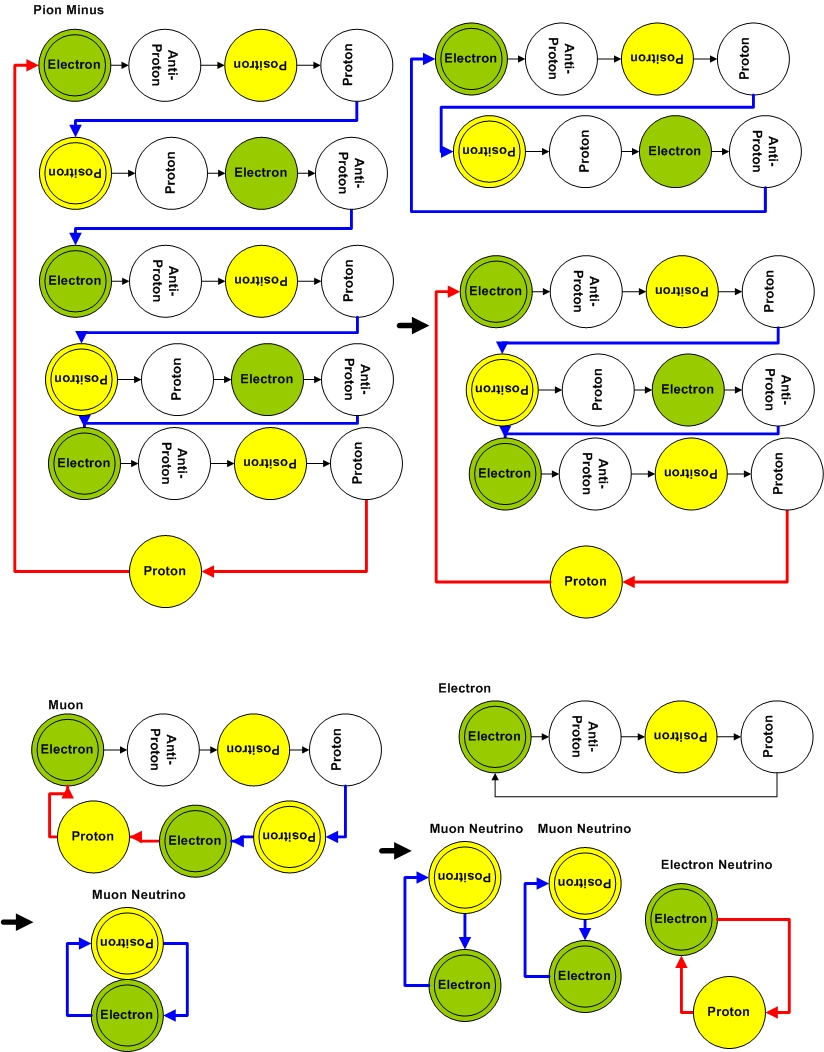Where here one would start with a pentameric pion minus (as opposed to a trimeric moiety) resulting in the expected neutrino ratio. This might be a better assignment for the pion state associated with the muon pathway. Current experiments are not sensitive enough to distinguish a trimeric from a pentameric pion. Assignments are tricky if one considers the lack of precision on the determination of the initial state of any decay reaction (e.g. Pion decay). Since the only discrepancy is the Pion Minus (trimeric) decaying into a muon Minus, I will consider that reaction to be starting from a Pion Minus* state (Pentameric Pion Minus).

The Muon Minus will have to be reassigned into: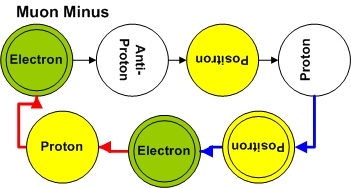Experiments that would probe the deformation susceptibility of the Fabric of Space are eons in the future - if not longer...:) They would be happening now if there wasn't such a horrendous censorship in Science.

Of course, those experiments would use the Coherent Fusion scheme I proposed...:)

Cheers,

MP

Currently unrated

### New Comment

required

required (not published)

optional

required